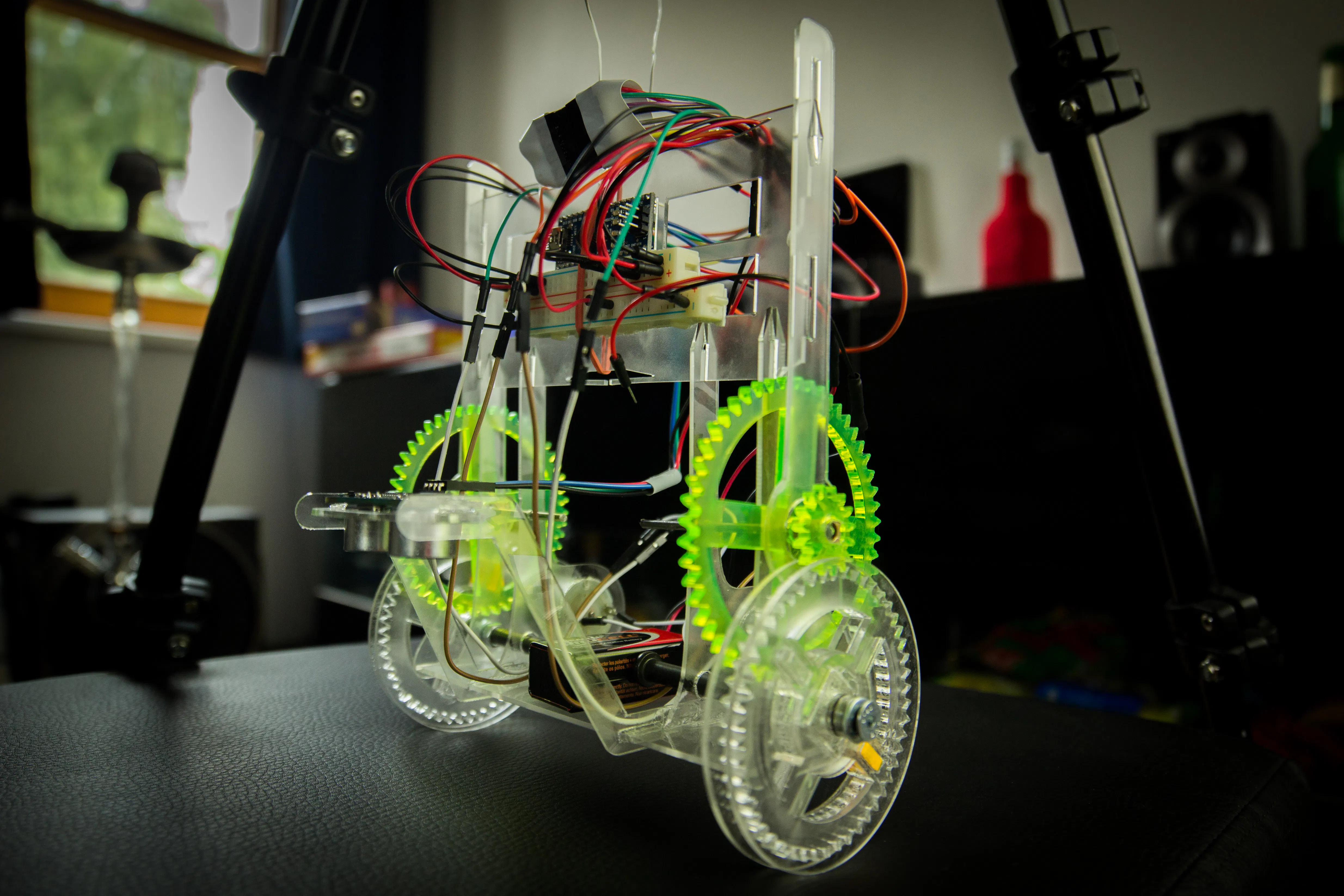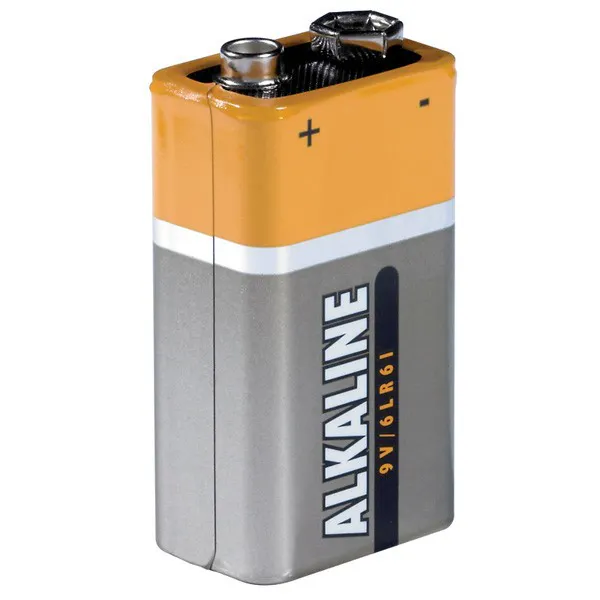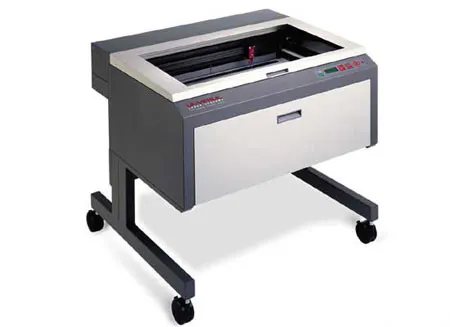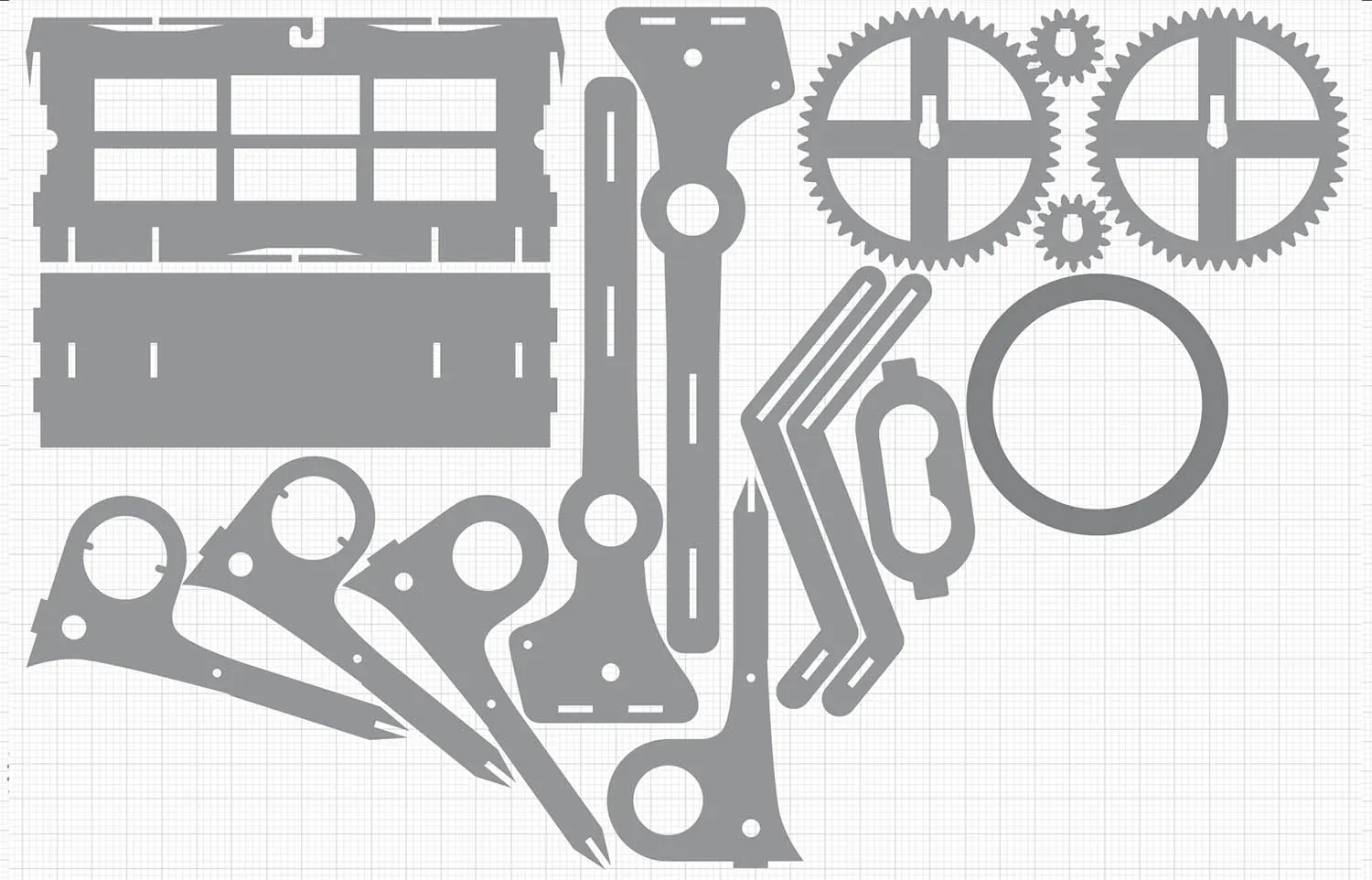# Self balancing robot

An autonomous robot created from laser-cuts, moving on two wheels and finding the brightest spot in a room.## Things used in this project

### Hardware componentsArduino Nano R3
×19V battery (generic)
×2

### Hand tools and fabrication machinesLaser cutter (generic)Soldering iron (generic)

## Custom parts and enclosures

### Laser-cut parts## Code

### BalanceRobot.ino

Arduino
Main code to control the robot movement
```#include <NewPing.h>

int internalLedPin = 13;
int externalLedPin = 12;
int potentiometerPin = A0;

int dc1EnablePin = 4;
int dc1Pin = 10;
int dc1ReversePin = 9;
int dc2EnablePin = 3;
int dc2Pin = 6;
int dc2ReversePin = 5;

int distanceTriggerPin = 8;
int distanceEchoPin = 7;
int maximumDistance = 5000;
int balancedDistanceOriginal = -1;
int balancedDistance = -1;

const int measuredDistancesCount = 10;
int measuredDistances[measuredDistancesCount];

int potentiometerValue = 512;

unsigned long timerLogLast = 0;
int timerLogDelta = 200;

unsigned long timerDistanceLast = 0;
int timerDistanceDelta = 10;

unsigned long timerDebugLast = 0;
int timerDebugDelta = 3000;

bool isTurningCW = true;

// magic numbers for power calculation
const float powerFactor0 = 0;
const float powerFactor2 = 0.00005;

NewPing sonar(distanceTriggerPin, distanceEchoPin, maximumDistance);

void setup() {
digitalWrite(internalLedPin, HIGH);
//pinMode(externalLedPin, OUTPUT);

pinMode(dc1EnablePin, OUTPUT);
pinMode(dc1Pin, OUTPUT);
pinMode(dc1ReversePin, OUTPUT);
pinMode(dc2EnablePin, OUTPUT);
pinMode(dc2Pin, OUTPUT);
pinMode(dc2ReversePin, OUTPUT);

pinMode(distanceTriggerPin, OUTPUT);
pinMode(distanceEchoPin, INPUT);
Serial.begin(9600);
}

void loop() {
unsigned long timerNow = millis();

// check sonar value
if (timerNow > timerDistanceLast + timerDistanceDelta) {
sonar.ping_timer(sonarCallback);
timerDistanceLast = timerNow;

// balance
double stableDistance = getAverageDistance(3);
if (stableDistance > balancedDistance + 1) {
//powerBothWheels(255);
} else if (stableDistance < balancedDistance - 1) {
//powerBothWheels(-255);
}
}

// debug
if (timerNow > timerDebugLast + timerDebugDelta) {

// change direction

if (isTurningCW) {
//powerBothWheels(-255);
} else {
//powerBothWheels(255);
}
isTurningCW = !isTurningCW;
Serial.println("Changing direction");

int powerValue = digitalToAnalog(potentiometerValue);

timerDebugLast = timerNow;
}

// log misc. stuff
if (timerNow > timerLogLast + timerLogDelta) {
// update potentiometer value

potentiometerValue = 512;
balancedDistance = balancedDistanceOriginal + (map(potentiometerValue - 512, 0, 512, 0, 10));

Serial.print("Last distances: ");
Serial.print(getMeasuredDistancesAsString());

Serial.print(" Average distance: ");
Serial.print(getAverageDistance(10));

Serial.print(" Last power value: ");
Serial.println(calculatePowerValue());

timerLogLast = timerNow;
}
}

void sonarCallback() {
if (sonar.check_timer()) {
int currentMeasuredDistance = (sonar.ping_result / 24);

// shift all elemts of the array by -1
for (char i = 0; i < measuredDistancesCount - 1; i++) {
measuredDistances[i] = measuredDistances[i + 1];
}

// store the new distance at the end of the array
measuredDistances[measuredDistancesCount - 1] = currentMeasuredDistance;

// if no balanced distance has been set, use the current one
if (balancedDistanceOriginal < 0) {
balancedDistanceOriginal = currentMeasuredDistance;
balancedDistance = balancedDistanceOriginal;
}

// let LED indicate if balanced or not
int deltaFromBalanced = currentMeasuredDistance - balancedDistance;

if (deltaFromBalanced <= 1 && deltaFromBalanced >= -1) {
digitalWrite(internalLedPin, HIGH);
} else {
digitalWrite(internalLedPin, LOW);
}

int powerValue = calculatePowerValue();
powerBothWheels(powerValue);

} else {
// invalid distance measured
}
}

int calculatePowerValue() {
int powerValue = 0;
double lastMeasuredDistance = measuredDistances[measuredDistancesCount - 1];
//double lastMeasuredDistance = getAverageDistance(5);

double deltaFromBalanced = lastMeasuredDistance - balancedDistance;

// modify power value using pedometer (centered pedometer --> factor = 1)
//double factor = map(potentiometerValue, 0, 1023, 0, 200) / (double) 100;
double factor = 1;

if (lastMeasuredDistance - balancedDistance < 0) {
deltaFromBalanced = deltaFromBalanced * (-1);
}

// powerValue is this formular solved for x:
// deltaFromBalanced = powerFactor2 * x^2 + powerFactor1 * x + powerFactor0
powerValue = factor * sqrt((deltaFromBalanced - powerFactor0) / powerFactor2);

if (powerValue == 0) {
double stableDistance = getAverageDistance(5);
double stableDistanceDelta = stableDistance - balancedDistance;
powerValue = 0.5 * sqrt((stableDistanceDelta) / powerFactor2);
if (stableDistanceDelta > 0) {
powerValue = powerValue * (-1);
}
} else {
if (deltaFromBalanced < 0) {
powerValue = powerValue * (-1);
}
}

powerValue = min(255, powerValue);
powerValue = max(-255, powerValue);

if (lastMeasuredDistance - balancedDistance > 0) {
powerValue = powerValue * (-1);
}

return powerValue;
}

double getAverageDistance(int count) {
if (count > 10) {
count = 10;
}

double average = 0;
for (char i = measuredDistancesCount - 1; i >= 0 && i + count >= measuredDistancesCount; i--) {
average += measuredDistances[i];
}

average = average / count;
return average;
}

String getMeasuredDistancesAsString() {
String distanceArray = "[";
for (char i = 0; i < measuredDistancesCount; i++) {
distanceArray = distanceArray + " " + measuredDistances[i] + " ";
}
distanceArray = distanceArray + "]";
return distanceArray;
}

void powerBothWheels(int value) {
powerLeftWheel(value);
powerRightWheel(value);
}

// Left wheel
void powerLeftWheel(int value) {
if (value > 0) {
powerLeftWheelForwards(value);
} else if (value < 0) {
powerLeftWheelBackwards(value * (-1));
} else {
stopLeftWheel();
}
}

void powerLeftWheelForwards(int value) {
digitalWrite(dc1EnablePin, HIGH);
analogWrite(dc1Pin, value);
analogWrite(dc1ReversePin, 0);
}

void powerLeftWheelBackwards(int value) {
digitalWrite(dc1EnablePin, HIGH);
analogWrite(dc1Pin, 0);
analogWrite(dc1ReversePin, value);
}

void stopLeftWheel() {
digitalWrite(dc1EnablePin, LOW);
analogWrite(dc1Pin, 0);
analogWrite(dc1ReversePin, 0);
}

// Right wheel
void powerRightWheel(int value) {
if (value > 0) {
powerRightWheelForwards(value);
} else if (value < 0) {
powerRightWheelBackwards(value * (-1));
} else {
stopRightWheel();
}
}

void powerRightWheelForwards(int value) {
digitalWrite(dc2EnablePin, HIGH);
analogWrite(dc2Pin, value);
analogWrite(dc2ReversePin, 0);
}

void powerRightWheelBackwards(int value) {
digitalWrite(dc2EnablePin, HIGH);
analogWrite(dc2Pin, 0);
analogWrite(dc2ReversePin, value);
}

void stopRightWheel() {
digitalWrite(dc2EnablePin, LOW);
analogWrite(dc2Pin, 0);
analogWrite(dc2ReversePin, 0);
}

int digitalToAnalog(int digitalValue) {
return digitalValue / 4;
}
```

## Credits

### Stephan Schultz

1 project • 10 followers
I develop apps: http://steppschuh.net